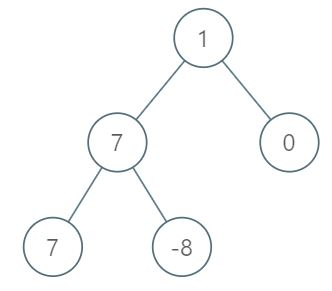# 1161. Maximum Level Sum of a Binary Tree

Given the `root` of a binary tree, the level of its root is `1`, the level of its children is `2`, and so on.

Return the smallest level `X` such that the sum of all the values of nodes at level `X` is maximal.

Example 1:Input:

```[1,7,0,7,-8,null,null]
```

Output:

```2
```

Explanation:

```Level 1 sum = 1.
Level 2 sum = 7 + 0 = 7.
Level 3 sum = 7 + -8 = -1.
So we return the level with the maximum sum which is level 2.
```

Note:

1. The number of nodes in the given tree is between `1` and `10^4`.
2. `-10^5 <= node.val <= 10^5`

I’m using level order traversal (BFS) here.

```/**
* Definition for a binary tree node.
* public class TreeNode {
*     int val;
*     TreeNode left;
*     TreeNode right;
*     TreeNode(int x) { val = x; }
* }
*/
class Solution {
public int maxLevelSum(TreeNode root) {
int max = 0;
int lv = 1;

int max_lv = 0;

while (! q.isEmpty()){

int size = q.size();
int level_sum = 0;
for (int i = 0; i < size; i ++){
TreeNode cur = q.poll();
if(cur.left != null)
if (cur.right != null)
level_sum += cur.val;
}
if (level_sum > max){
max = level_sum;
max_lv = lv;
}
lv ++;

}
return max_lv;
}

}```